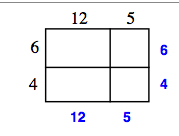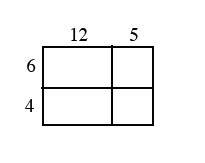### Home > AC > Chapter 4 > Lesson 4.2.2 > Problem4-83

4-83.

Use the rectangle below to answer the following questions.

1. Find the area of the entire rectangle. Explain how you found your solution.

• Find the area of the individual parts, then sum the parts.

• Find the total length of each side.

• Find the area of the large rectangle by multiplying the length of each side.

• 170 square units

1. Calculate the perimeter of the figure.

• Find the length of each side, then sum the sides.

•